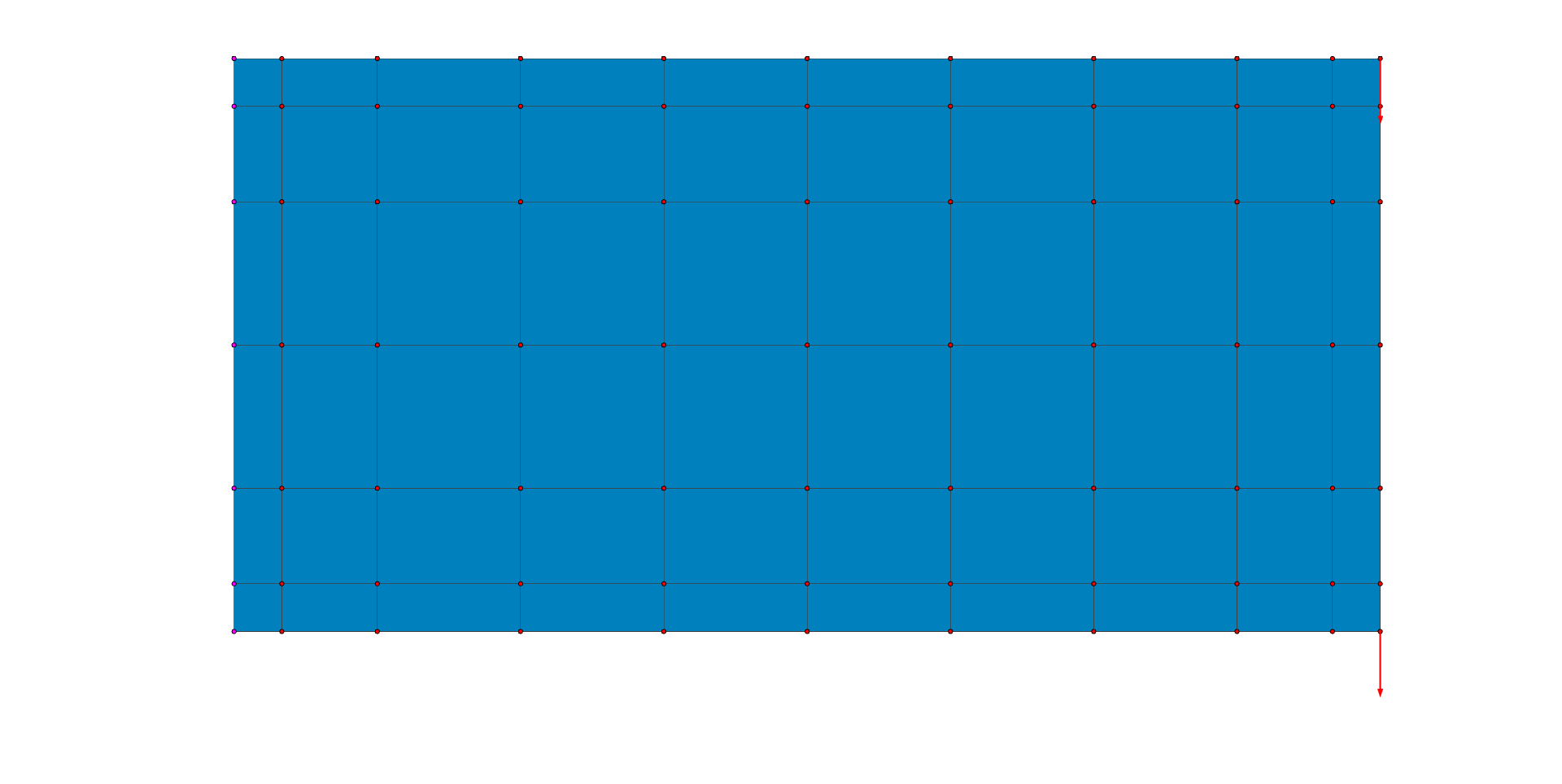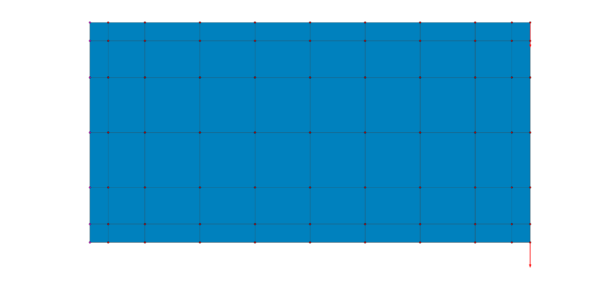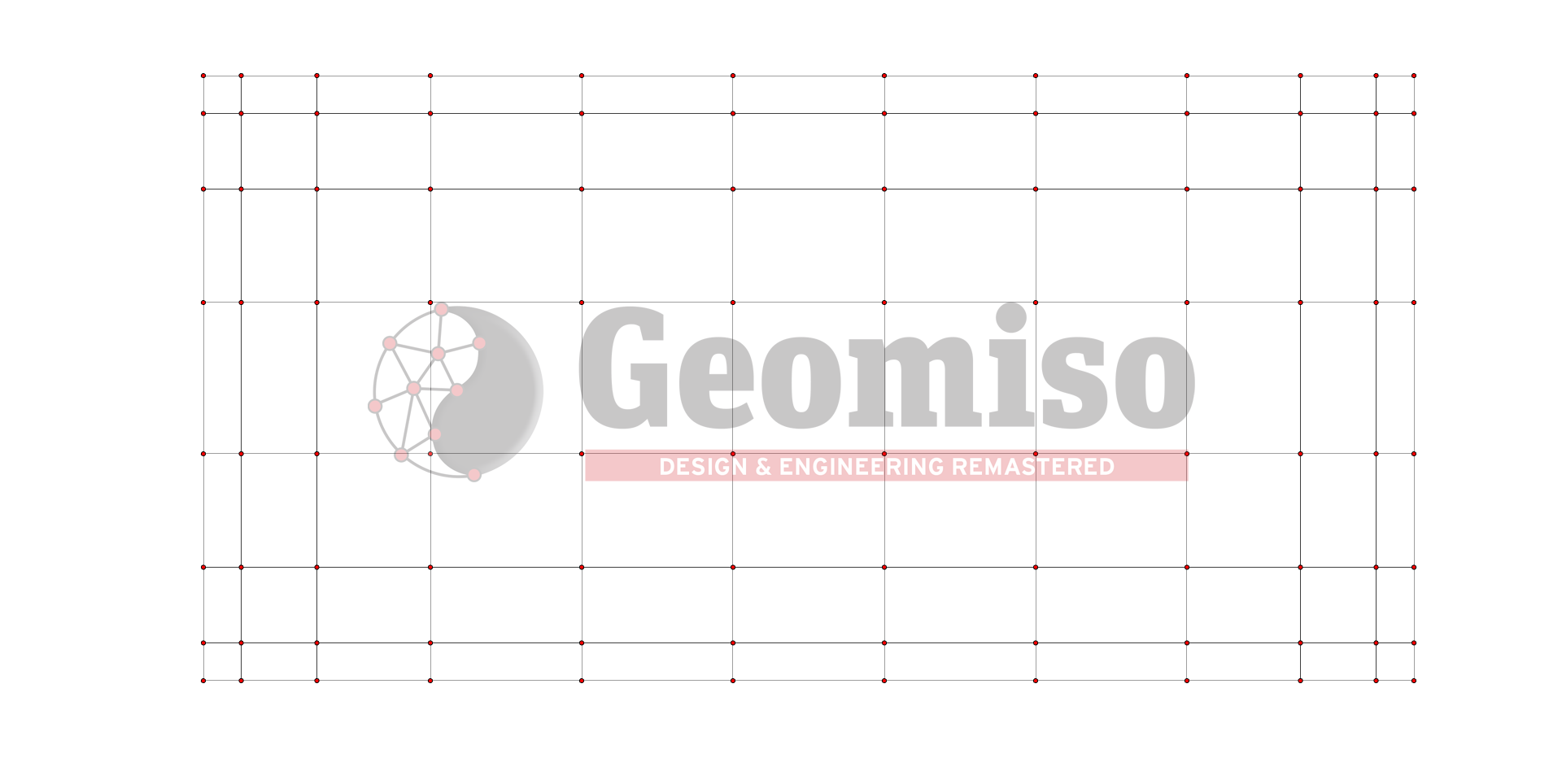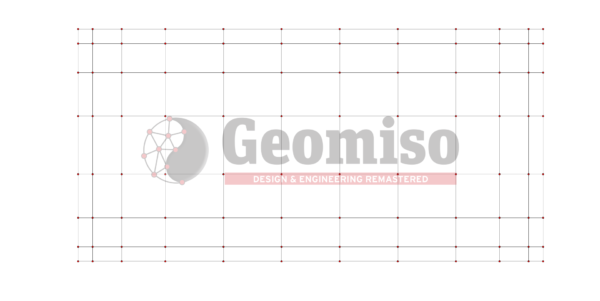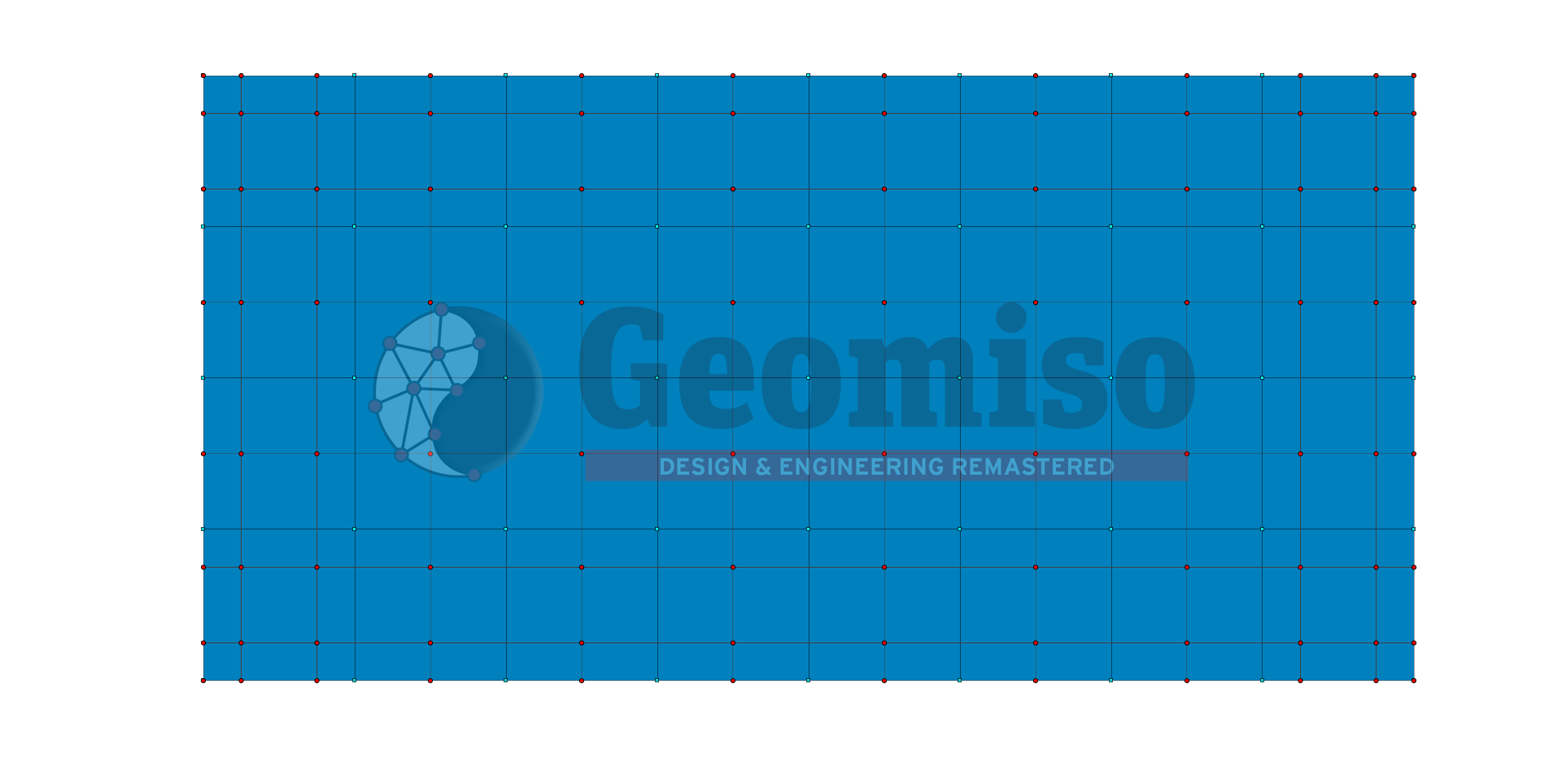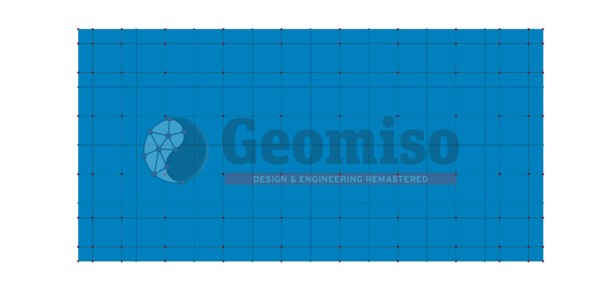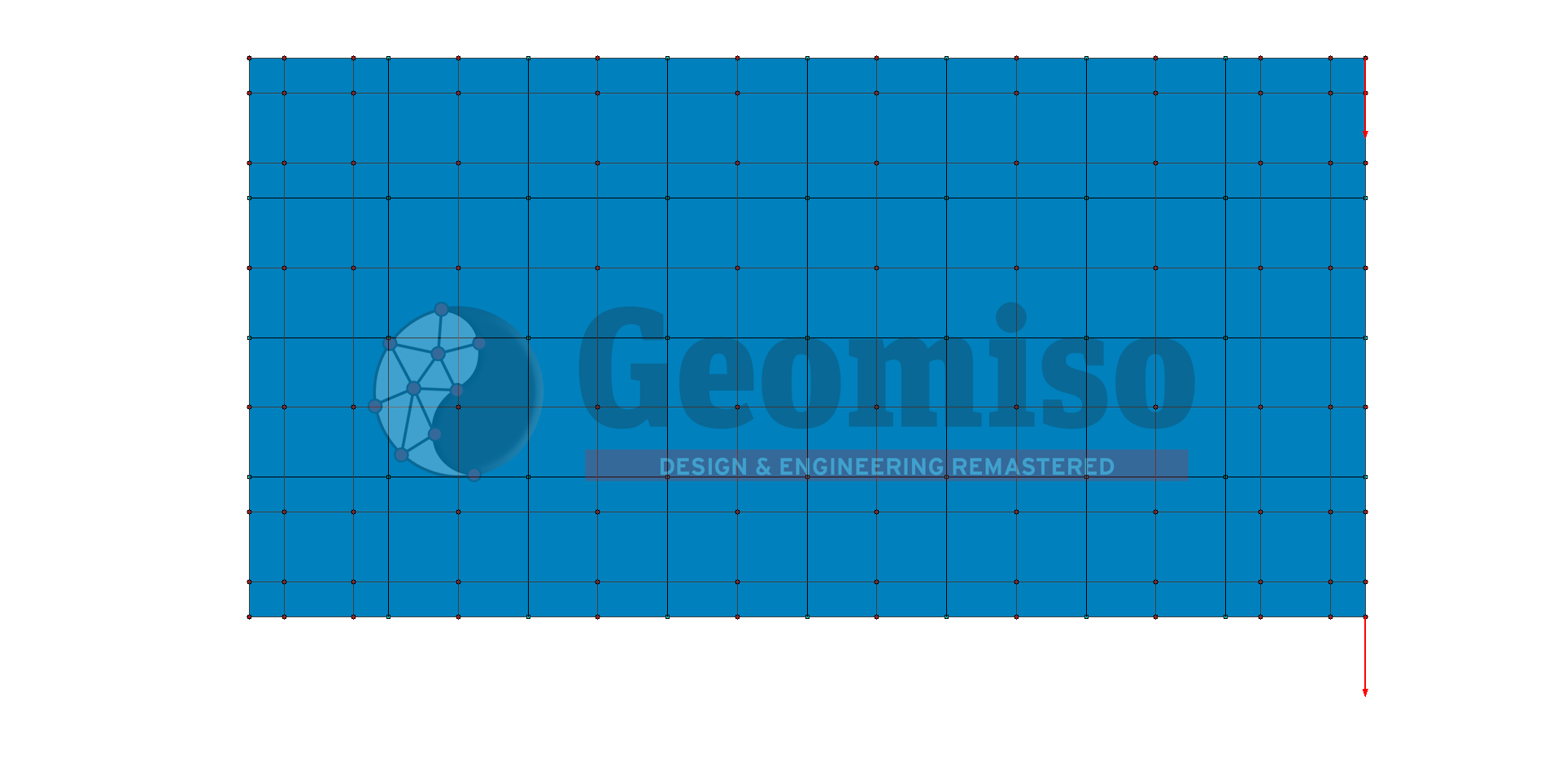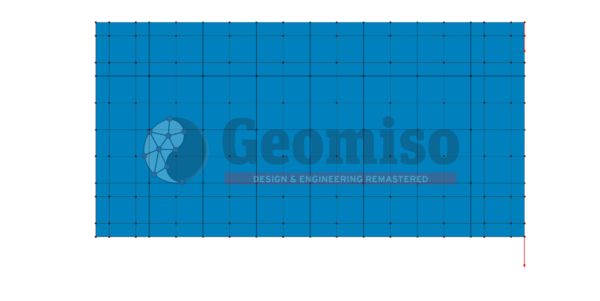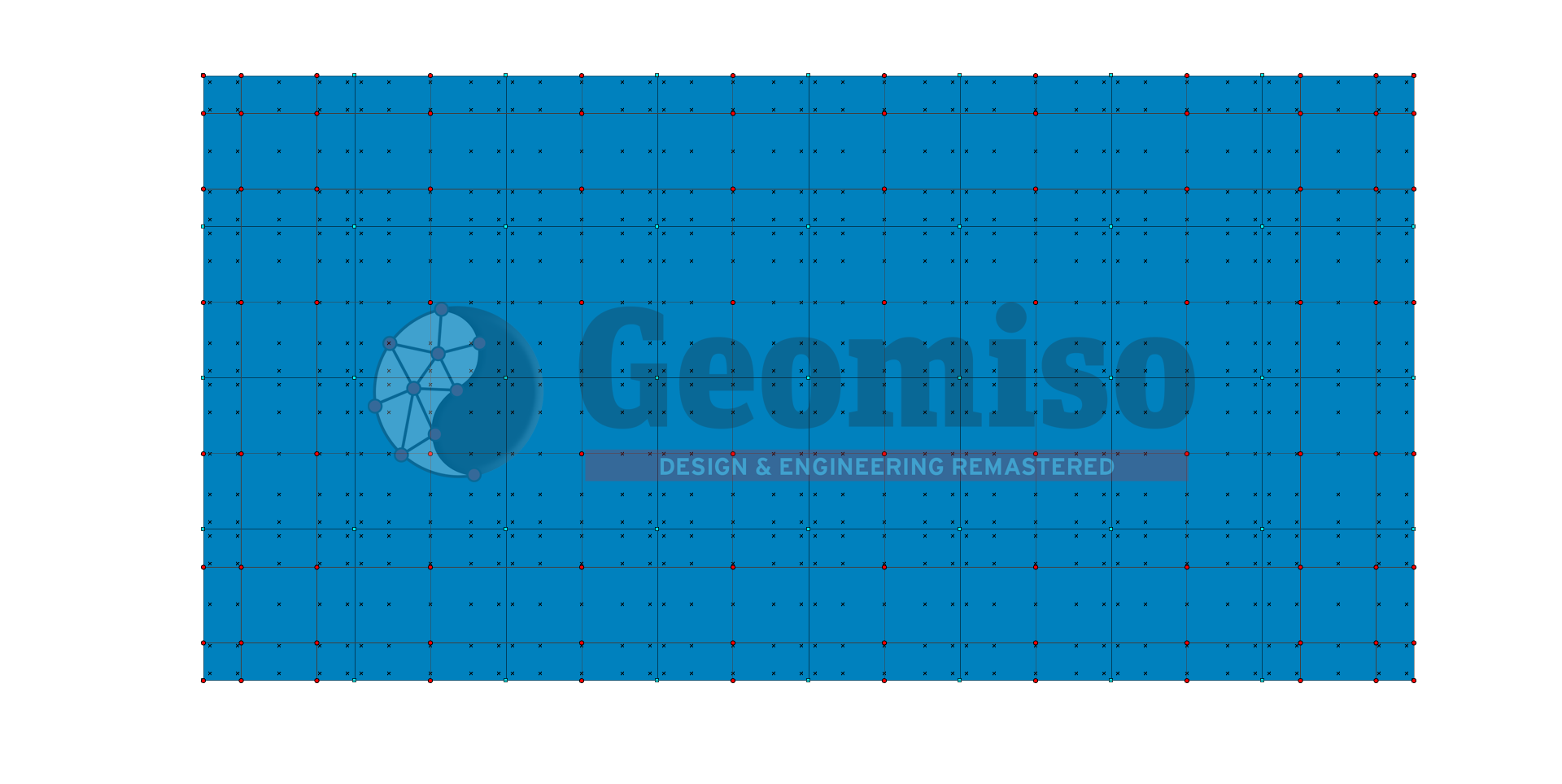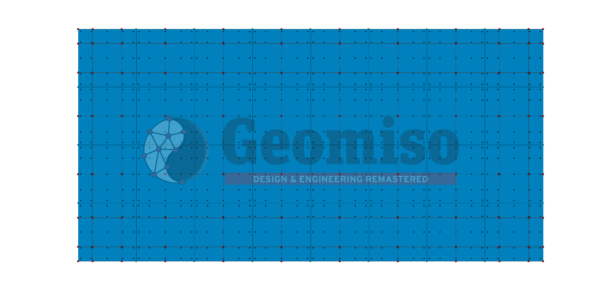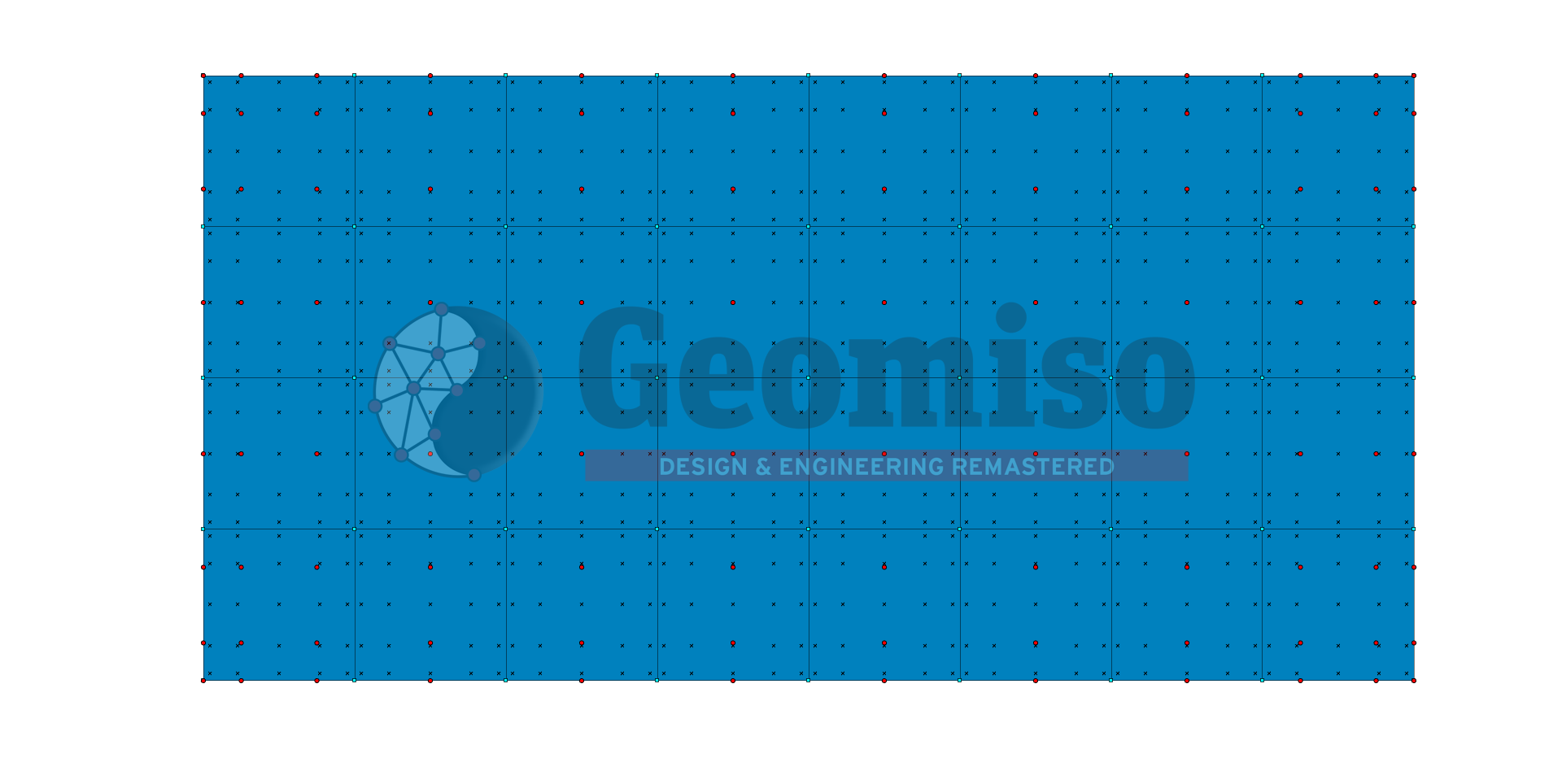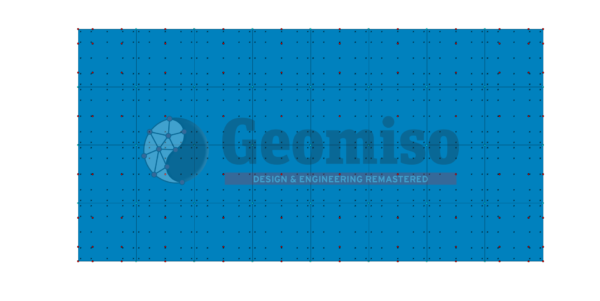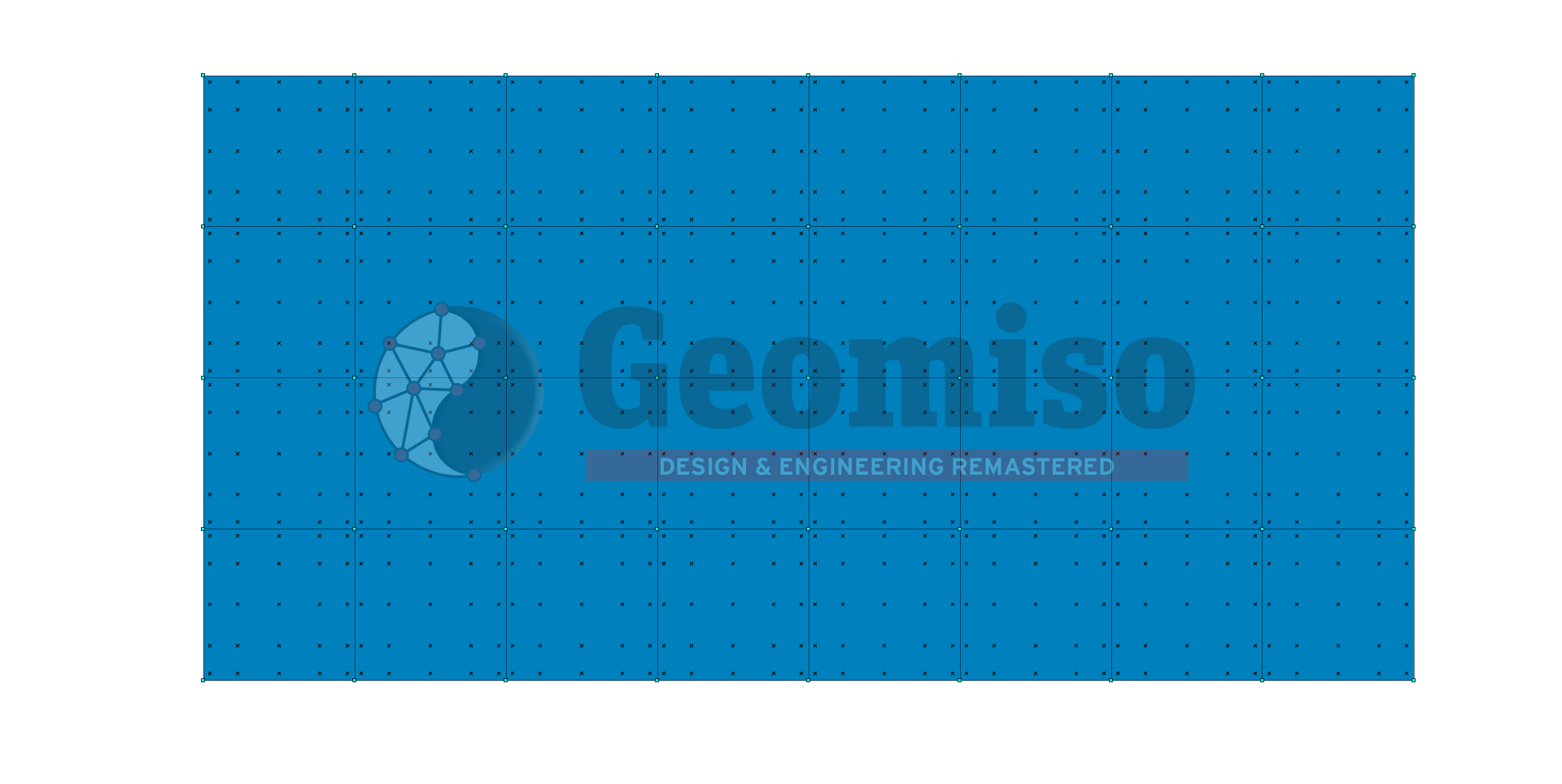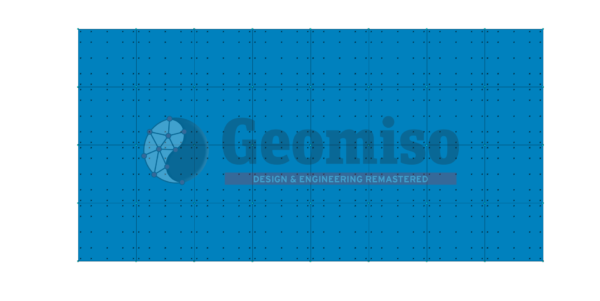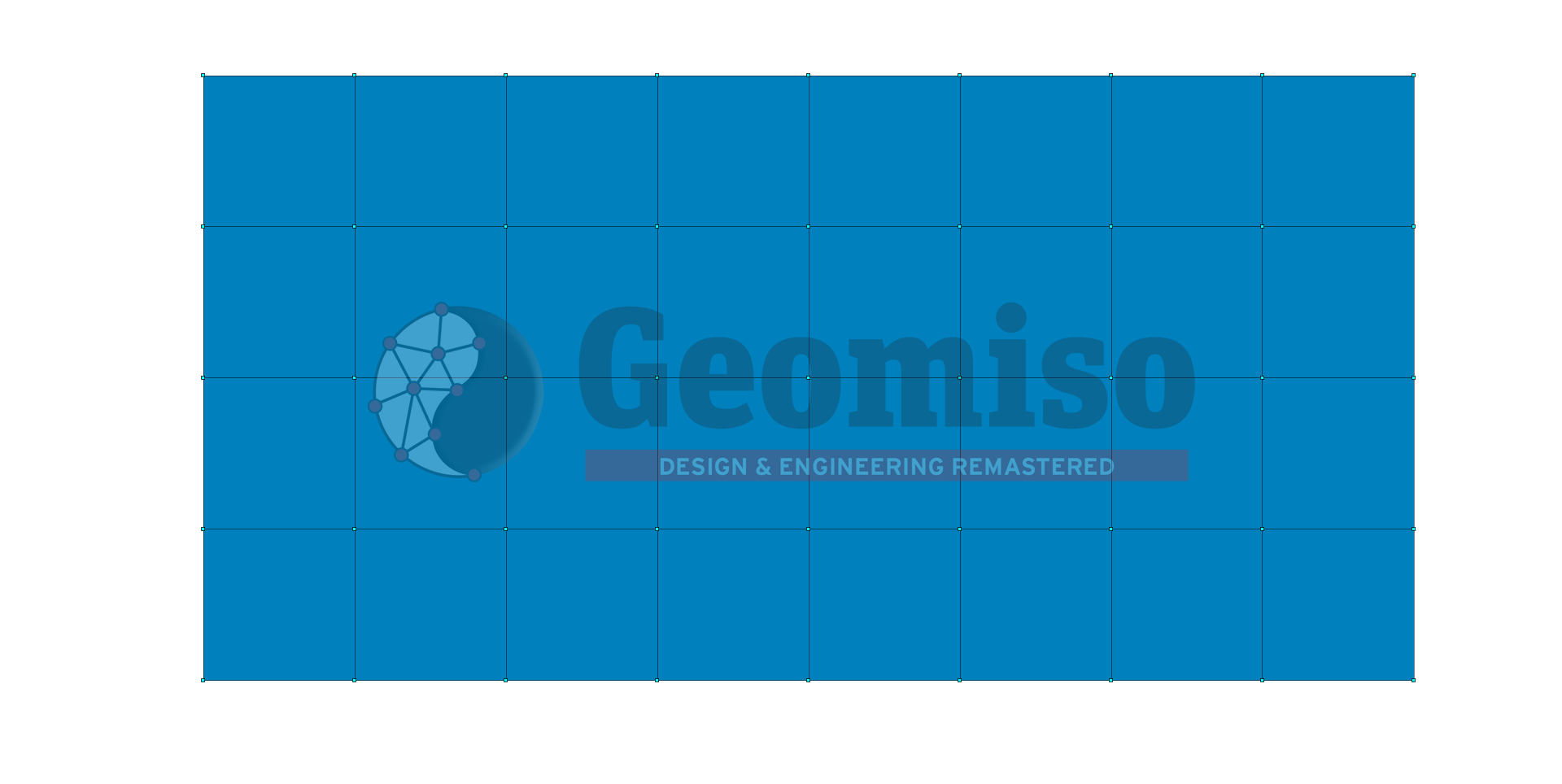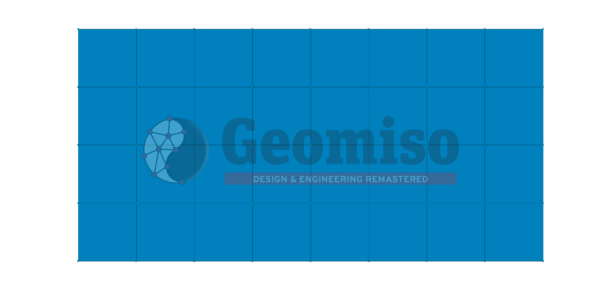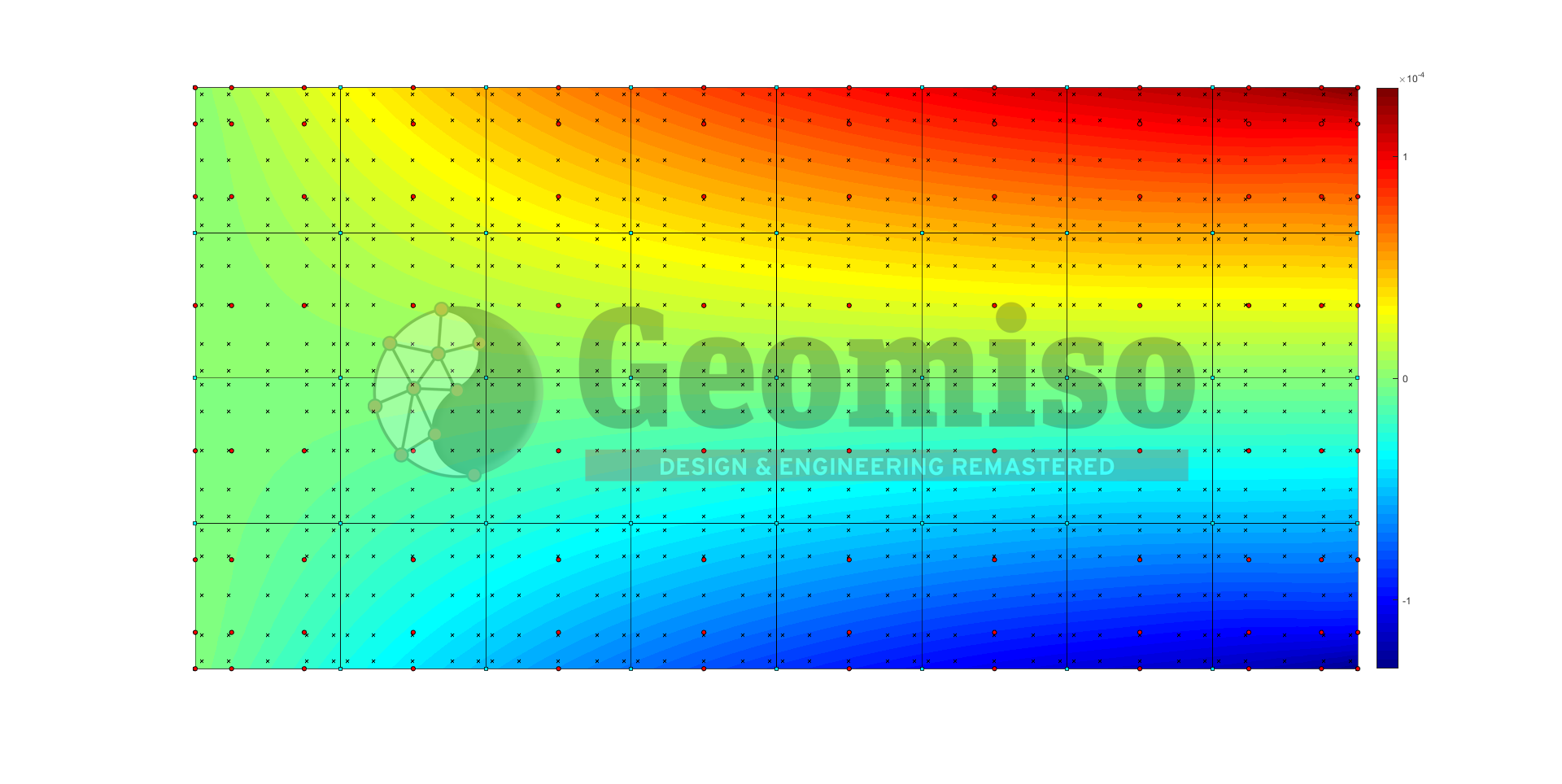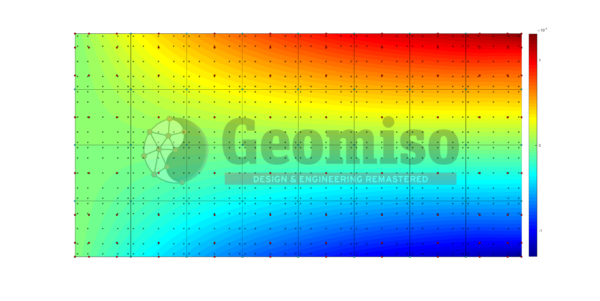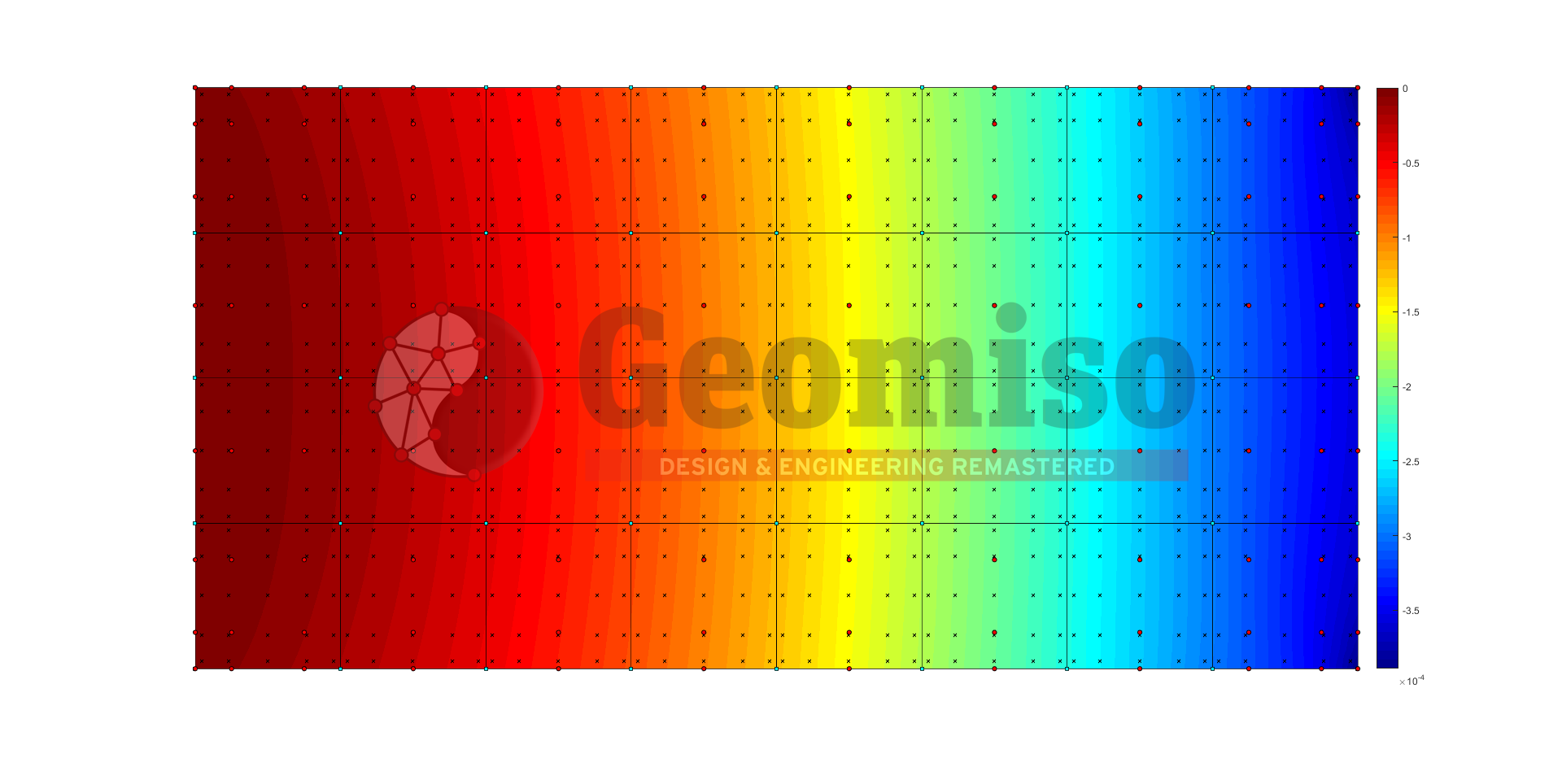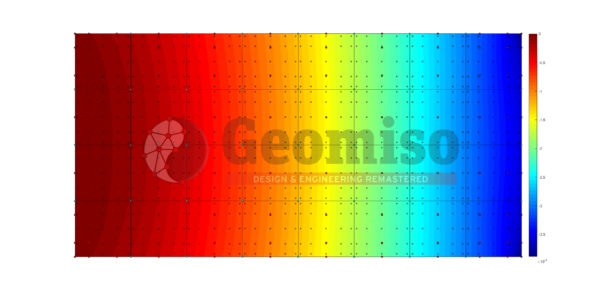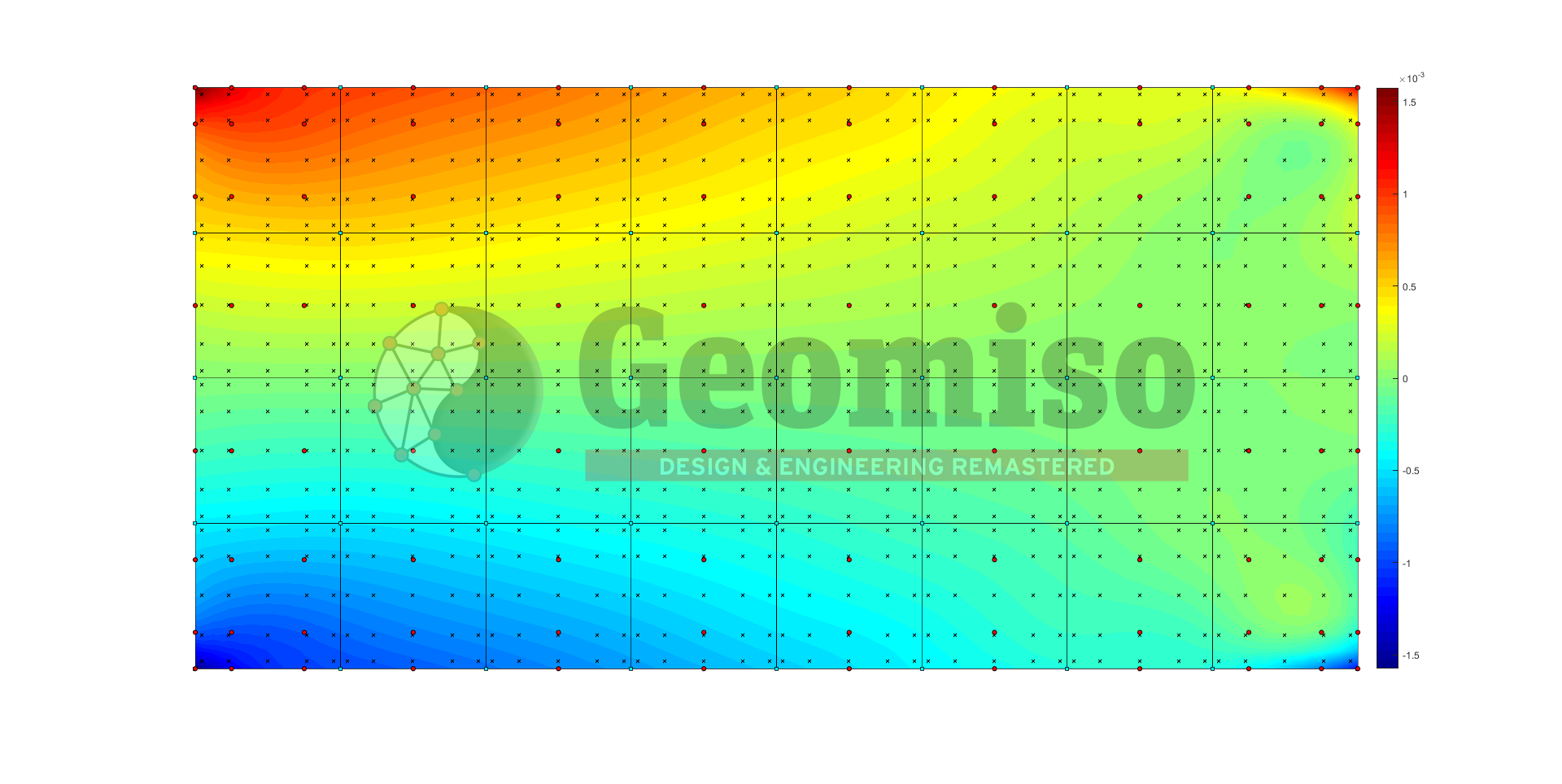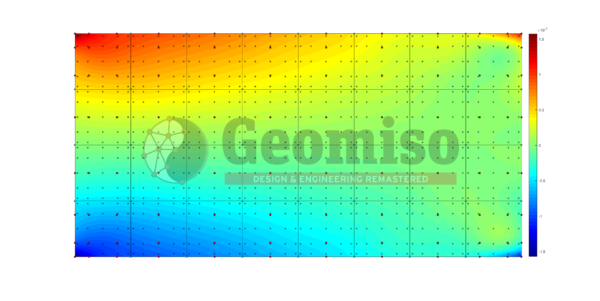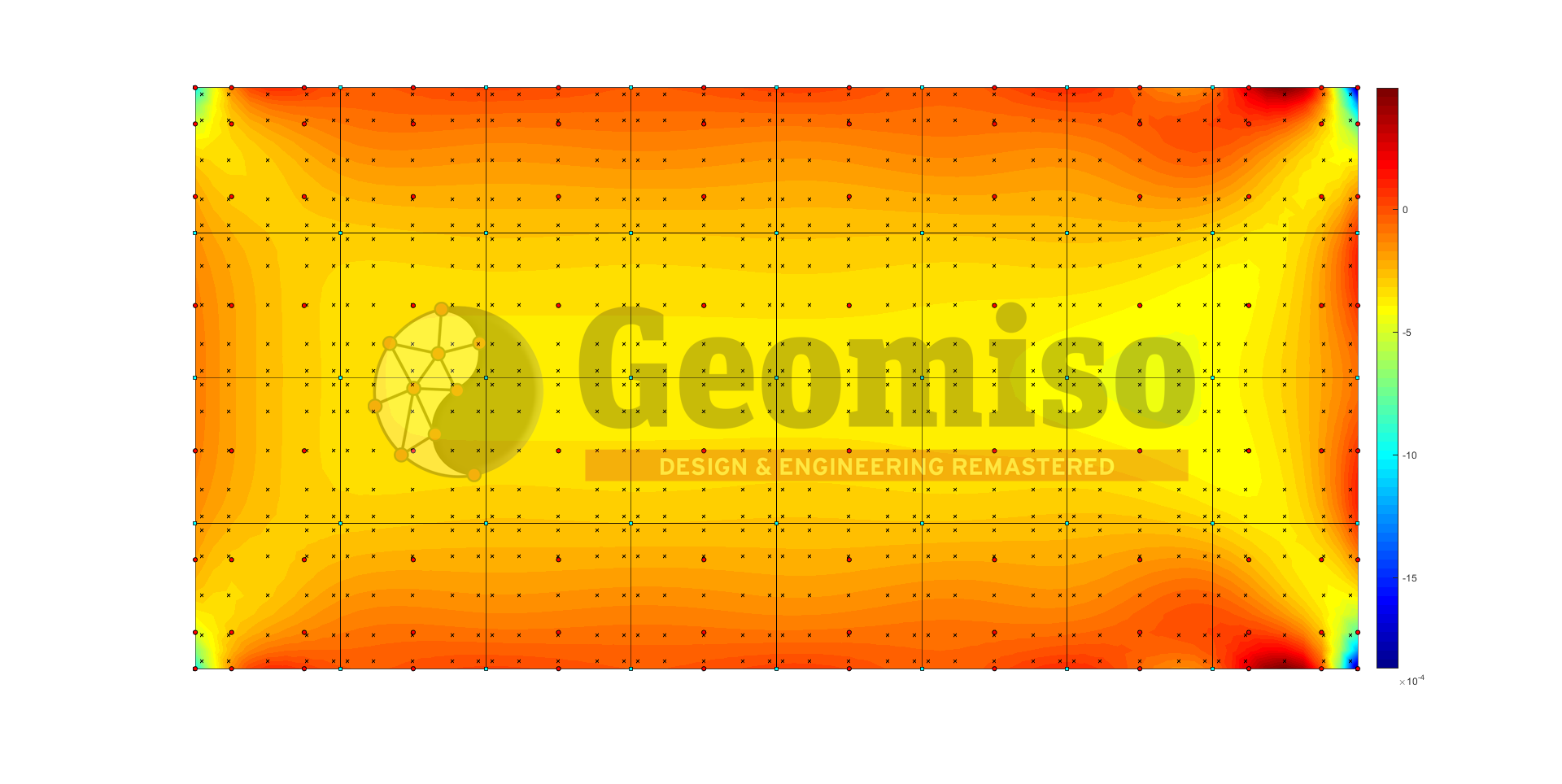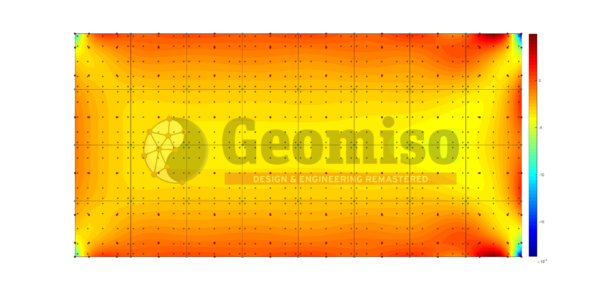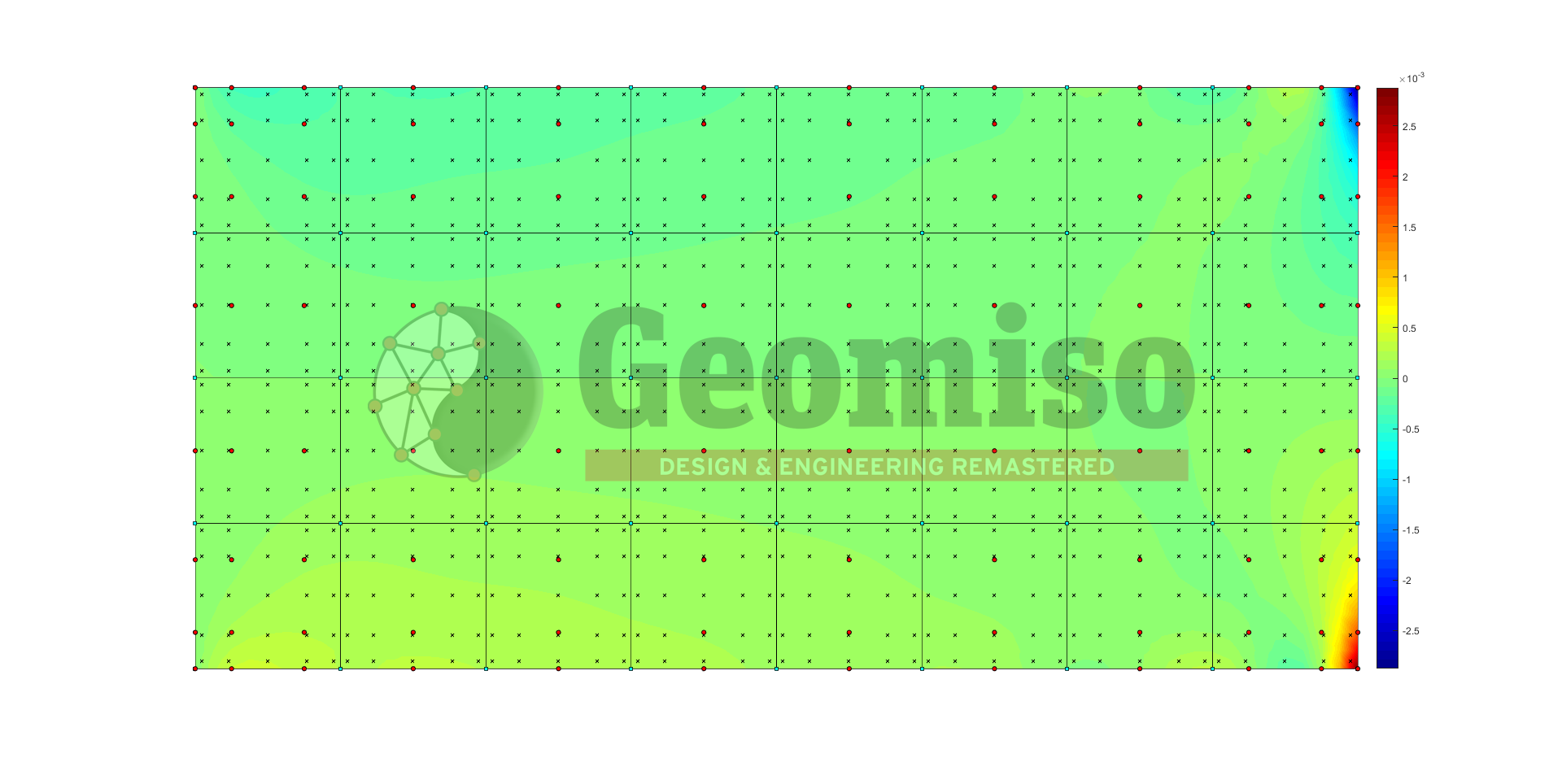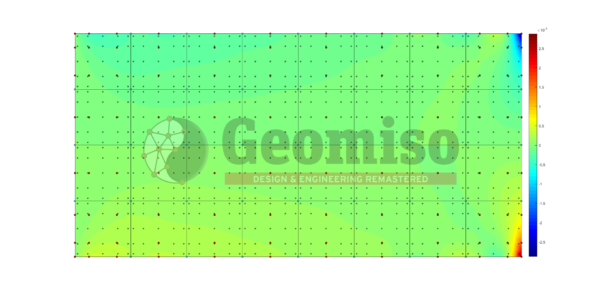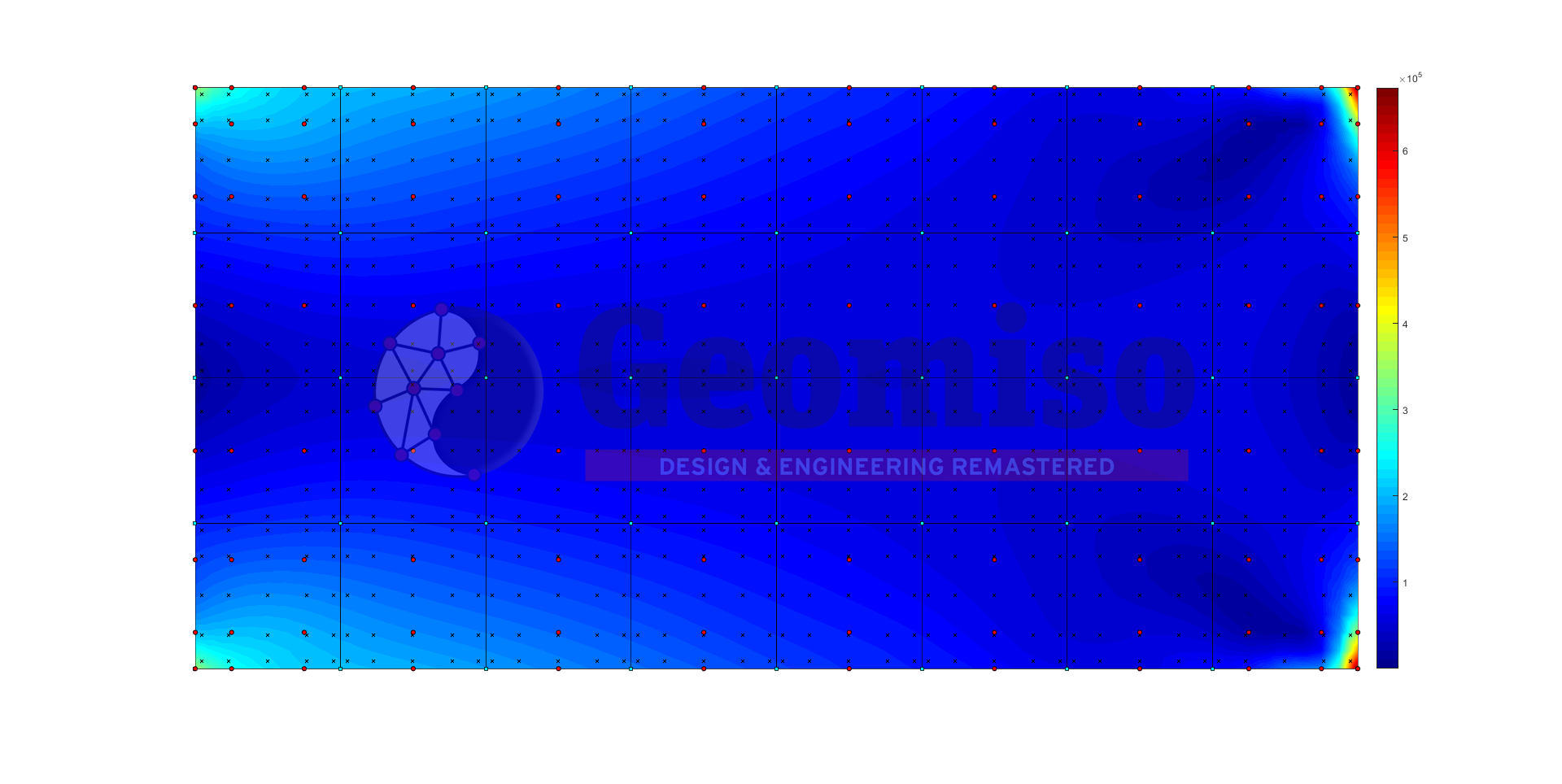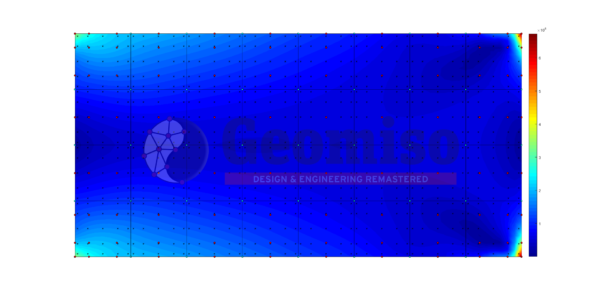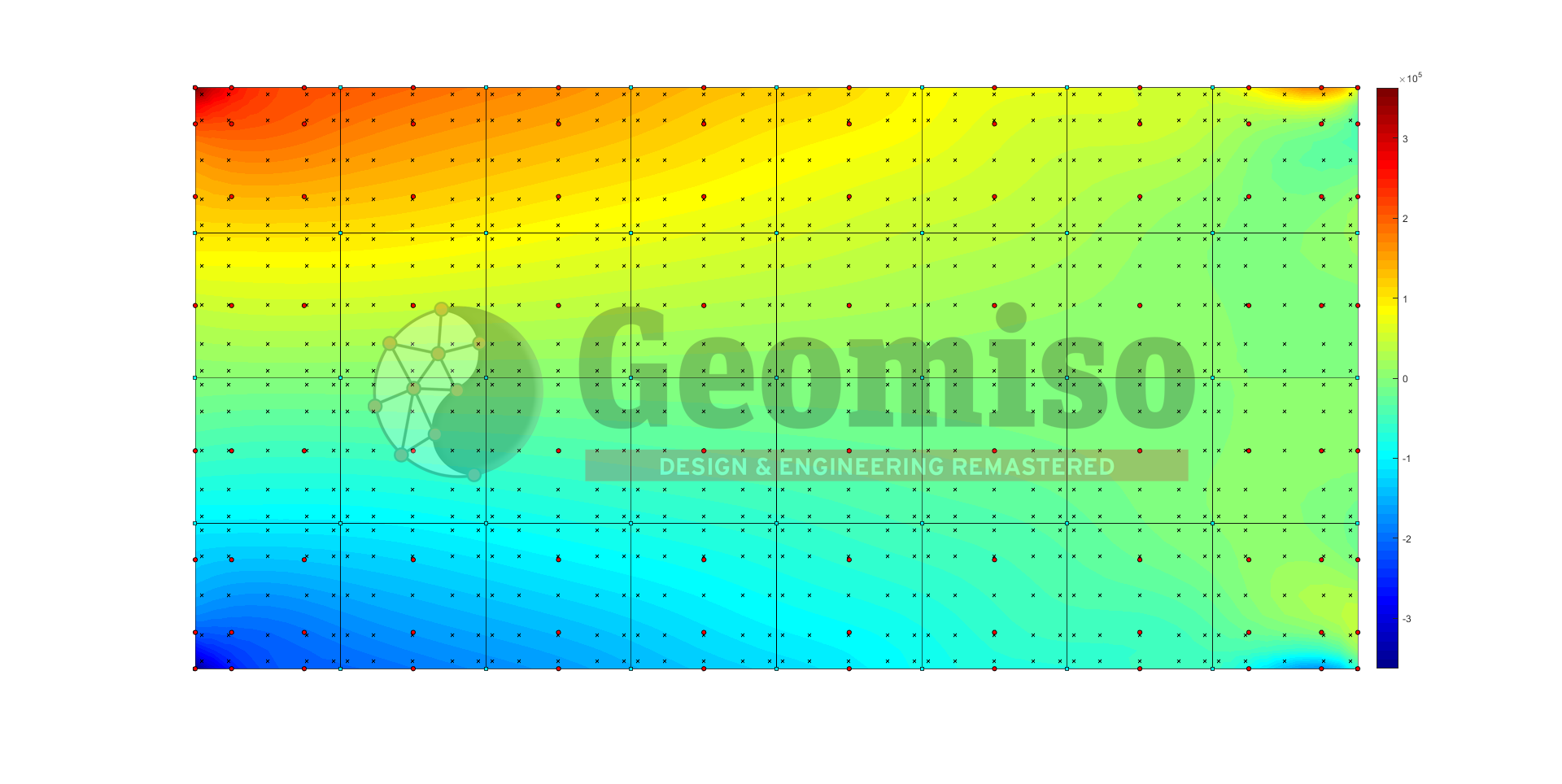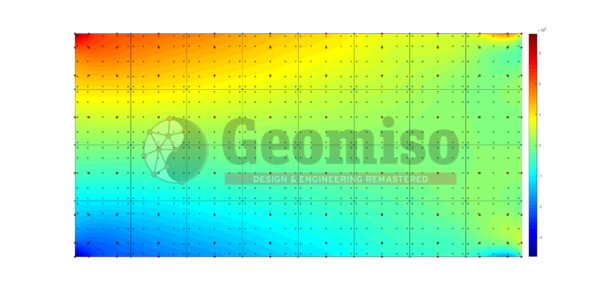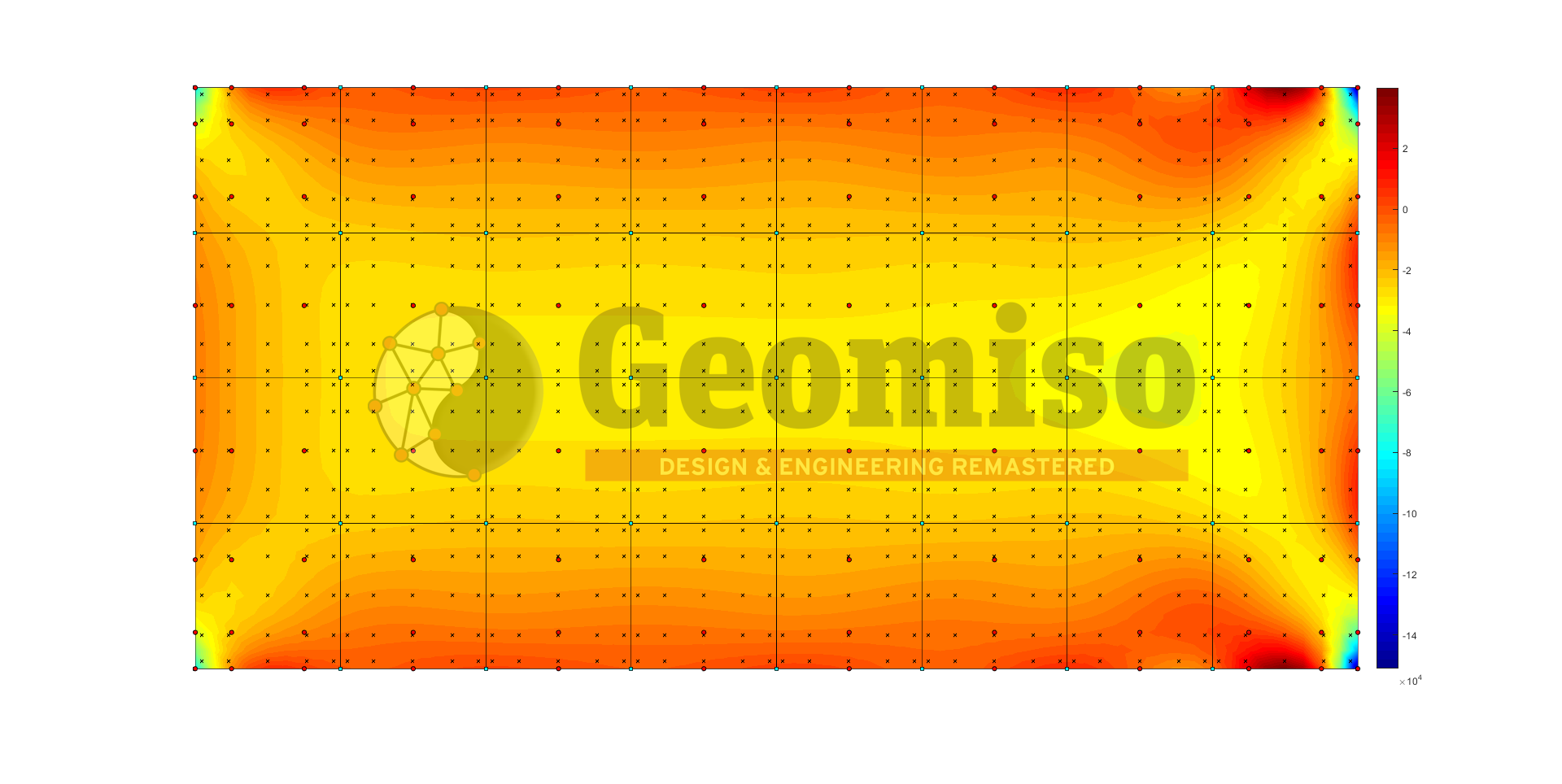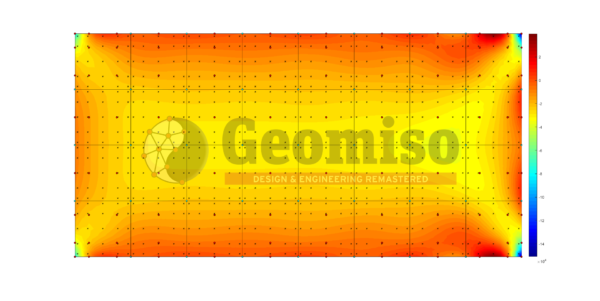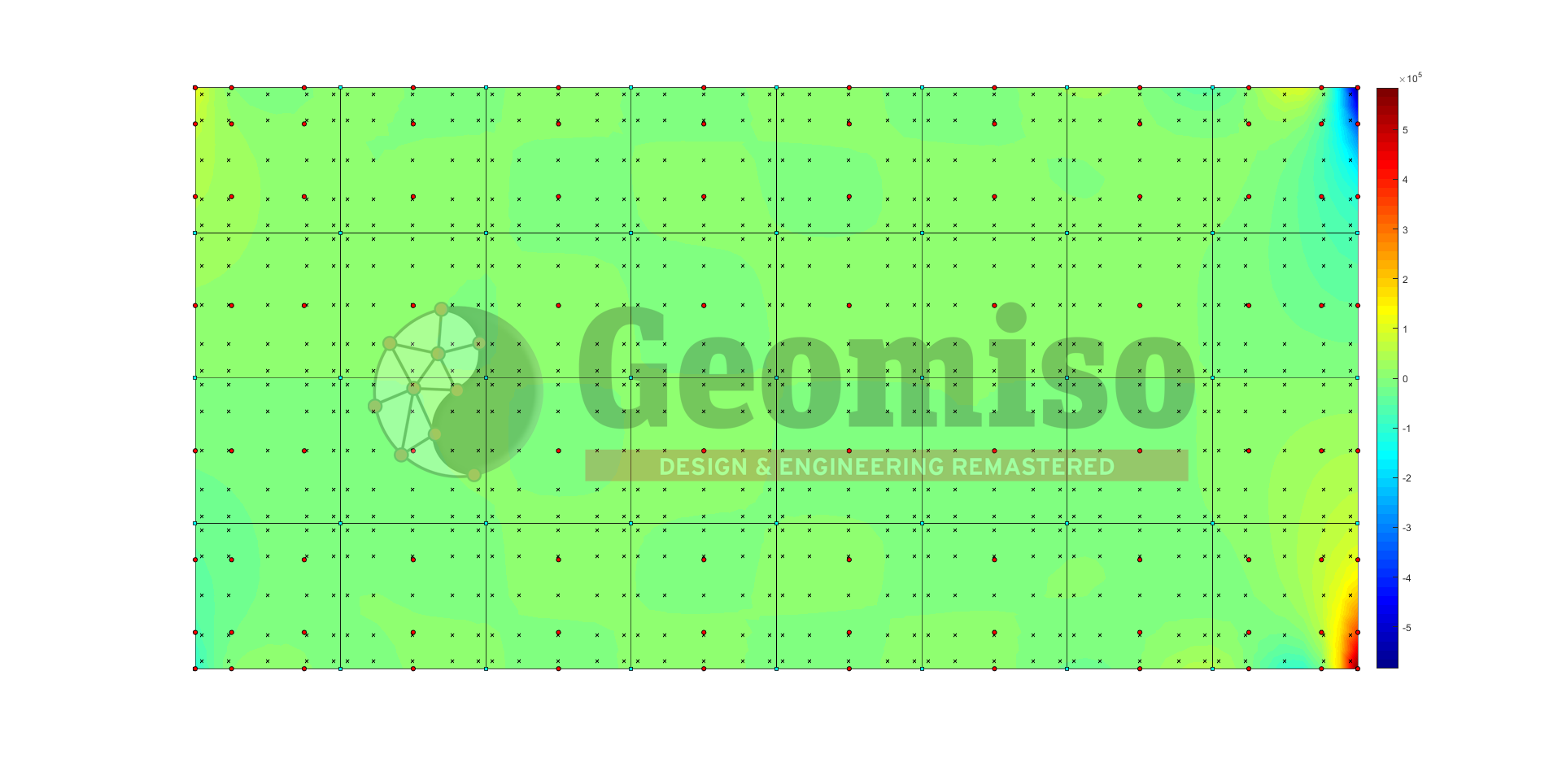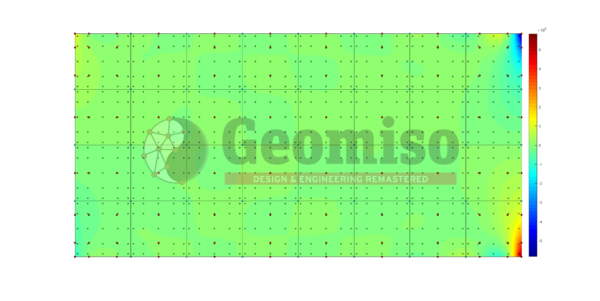# 130001 Metal Plate v1.0

99,99

This project presents the effect of both knot span number and basis polynomial order on the numerical solution of a cantilever metal plate with homogeneous Dirichlet boundary conditions, described with NURBS and subjected to concentrated loads.

Categories: ,

## Description

This project presents the effect of both knot span number and basis polynomial order on the numerical solution of a cantilever metal plate with homogeneous Dirichlet boundary conditions, described with NURBS and subjected to concentrated loads. This parametric investigation includes a set of 9 comparisons with a total number of 20 meshes submitted to linear elastic static NURBS-based isogeometric analysis using Geomiso. The results contain pseudo-displacements (control points), Cartesian coordinates (points, Gauss points) and displacement/ strain/ stress vectors (points, Gauss points). Error estimation is based on the finest mesh which is different for each comparison, while a comparison with the 3D model (140001) was conducted to estimate the error introduced by the plane stress assumptions.

Specifications
Product ID 130001
Published July 25, 2018
Size 30.2 MB
Product Type Project
Mesh Number 20
Geometry Rectangle
Material Steel
Constraint Dirichlet (homogeneous)
Load Concentrated
Analysis Type Isogeometric (NURBS) – Static – 2D
Material/ Geometry linearity Linear/ Linear
Quadrature Gauss-Legendre

This pack includes all the following files, which contain the control variables and the knot vectors required to produce the 20 NURBS models, the loading and the boundary conditions needed to solve the problem and the respective results of the 20 analyses (tables, charts, contours) obtained using Geomiso Terminator v1.0 software. Displacement and stress fields are compared between 2D and 3D analysis (140001) to estimate the errors introduced by the plane stress assumptions.

## Reviews

There are no reviews yet.